# Degenerate parabolic equation

(diff) ← Older revision | Latest revision (diff) | Newer revision → (diff)

A partial differential equation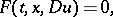where the functionhas the following property: For some even natural number, all rootsof the polynomial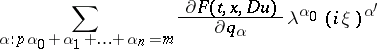have non-positive real parts for all realand, for certain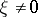,,, and,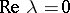for some root, or for certain,and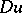the leading coefficient at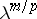vanishes. Hereis an independent variable which is often interpreted as time;is an-dimensional vector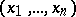;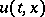is the unknown function;is a multi-index;is the vector with components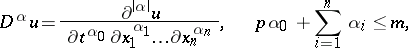is a vector with components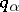,is an-dimensional vector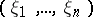, and. See also Degenerate partial differential equation, and the references given there.

How to Cite This Entry:
Degenerate parabolic equation. Encyclopedia of Mathematics. URL: http://encyclopediaofmath.org/index.php?title=Degenerate_parabolic_equation&oldid=18826
This article was adapted from an original article by A.M. Il'in (originator), which appeared in Encyclopedia of Mathematics - ISBN 1402006098. See original article